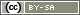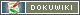[[putList()]]

# putList()

`void putList(string Question, array value, [boolean EachVariable])`

With the function `putList()` you can store the values of an (one-dimensional) Array into an array of variables in the data set. The function runs equivalent to `put()` and `valueList()`.

• Question
The identifier of the question of type “internal variable” (see below), in which the values are to be stored. The identifier is specified as a string in quotation marks, e.g. `'IV01`'. In the question, at least as many variables have to be created as the array has elements.
• values
An array with numbers or texts (strings), which should be stored in variables.
• EachVariable (optional)
Usually `putList` saves only in internal variables. In exceptional cases it may be useful to overwrite the values of other question types. In this case a third parameter `true` can be specified.

Warning: Only use the third parameter EachVariable if you know exactly what you are doing. If you change the answering code you can overwrite the answers of the responents.

## Internal Variables

Before `putList()` can be used, create a question of type Internal variables in the question catalog. In it you create at least as many variables (items) as the array element has that you want to save.

en/create/functions/putlist.txt · Last modified: 30.09.2021 10:26 by sophia.schauer